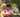### Dominator

February 01, 2020

Dominator

An array A consisting of N integers is given. The dominator of array A is the value that occurs in more than half of the elements of A.

For example, consider array A such that
A = 3 A = 4 A = 3
A = 2 A = 3 A = -1
A = 3 A = 3

The dominator of A is 3 because it occurs in 5 out of 8 elements of A (namely in those with indices 0, 2, 4, 6 and 7) and 5 is more than a half of 8.

Write a function
function solution(A);
that, given an array A consisting of N integers, returns index of any element of array A in which the dominator of A occurs. The function should return −1 if array A does not have a dominator.

For example, given array A such that
A = 3 A = 4 A = 3 A = 2 A = 3 A = -1 A = 3 A = 3 the function may return 0, 2, 4, 6 or 7, as explained above.

Write an efficient algorithm for the following assumptions:
• N is an integer within the range [0..100,000];
• each element of array A is an integer within the range [−2,147,483,648..2,147,483,647].

``````function solution(A) {
// write your code in JavaScript (Node.js 8.9.4)
if(A.length<1) return -1

A1 = A.slice()
A1.sort((a,b)=>a-b)
const h = Math.floor(A1.length/2) // middle index
const d = A1[h]

let res = [], count = 0
for(let i=0; i<A.length; i++){
if(A[i]==d){
res.push(i)
count ++
}
}
//console.log(res)
if( count > h ) return res
else return -1
}``````Posted by Tai Lu ( 呂台生 ) who's building useful things.You should follow him on Twitter